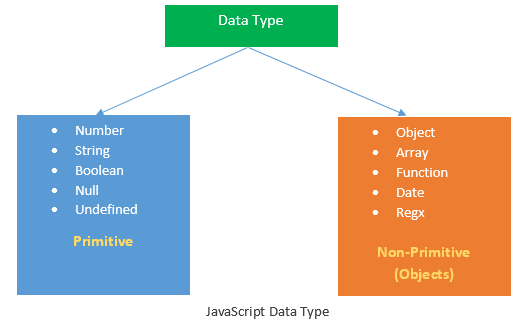# Understanding Datatypes in JavaScript

JavaScript is a dynamic programming language. It means you don't have to declare the type of a variable at the time of declaration, it's type will be determine automatically at the time of program execution i.e at run-time. This is the reason you can have the same variable for different types.

### Javascript Dynamic typing Example

```<script>
var x = 42; // here, x is a Number
var x = "DOT NET TRICKS"; // here, x is a String
var x = true; // here, x is a Boolean
var x = {name:'John', age:34}; // here, x is an Object
</script>
```

## JavaScript Data types

Typically, JavaScript has two types of data types : Primitive and Non-Primitive(Objects).## The typeof Operator

JavaScript typeof operator is used to find the type of a JavaScript variable.

```<script>
typeof "John" // returns string
typeof 3.14 // returns number
typeof Infinity; // returns number
typeof NaN; // returns number
typeof false // returns Boolean
typeof null // returns object and this is bug in ECMA script5

typeof [1,2,3,4] // returns object
typeof {name:'John', age:34} // returns object
typeof function(){} // returns function
typeof /^[0-9]\$/I // returns object

var d = new Date();
typeof d // returns object
</script>
```
##### What do you think?